### Home > CALC > Chapter 8 > Lesson 8.1.6 > Problem8-63

8-63.
1. At which point(s) on f(x), graph below, do the following situations occur? Homework Help ✎

2.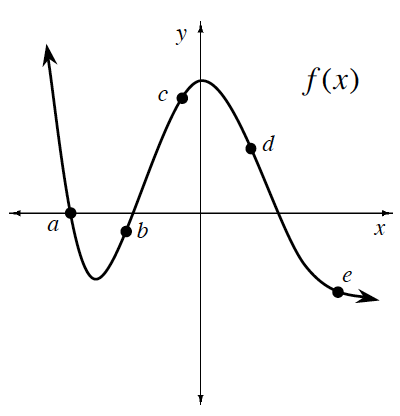1. f ′(x) and f ′′(x) are both positive

2. f ′(x) and f ′′(x) are both negative

3. f ′(x) is positive and f ′′(x) is negative

4. f ′(x) is negative and f ′′(x) is positive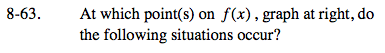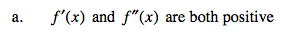Translation: slope of f(x) is positive AND f(x) is concave up.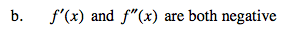Refer to the hint in part (a).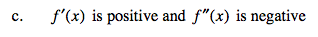Refer to the hint in part (a).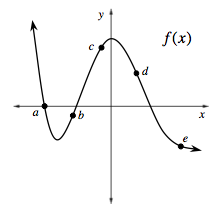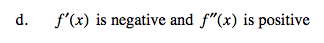Points e and a.